# 如何理解卷积--Understanding Convolutions

## 抛球实验 -- Ball drop experiment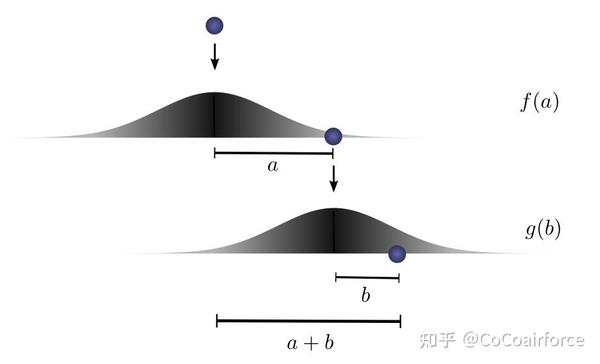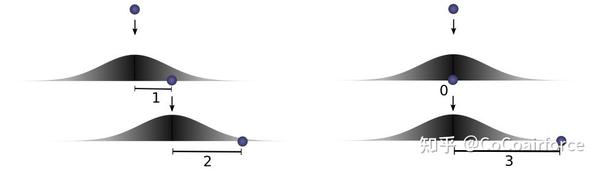$$\ldots f(0) \cdot g(3)+f(1) \cdot g(2)+f(2) \cdot g(1) \ldots$$

$$\sum_{a+b=c} f(a) \cdot g(b)$$

$f$ 和 $g$ 的卷积，在 $c$ 处被定义为。

$$(f * g)(c)=\sum_{a+b=c} f(a) \cdot g(b)$$

$$(f * g)(c)=\sum_{a} f(a) \cdot g(c-a)$$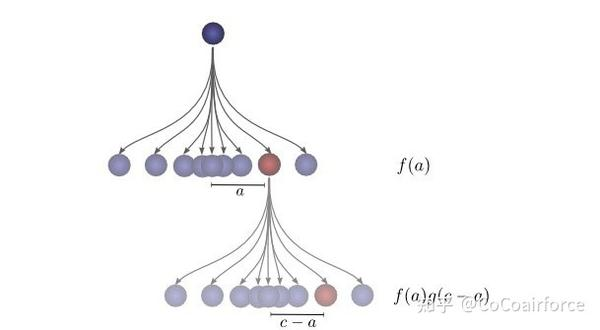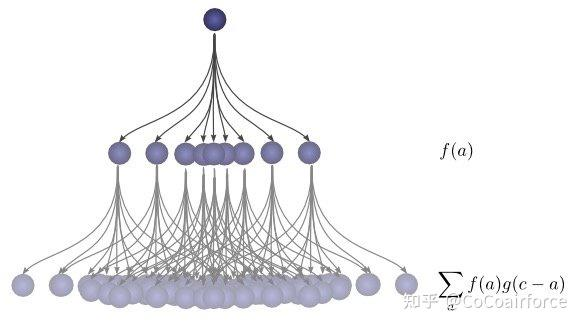## 可视化卷积 -- Visualizing Convolutions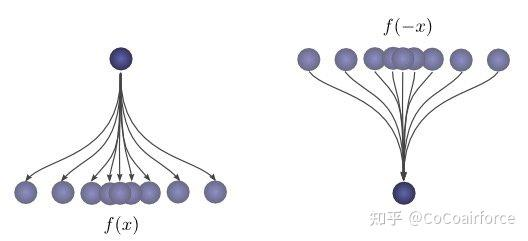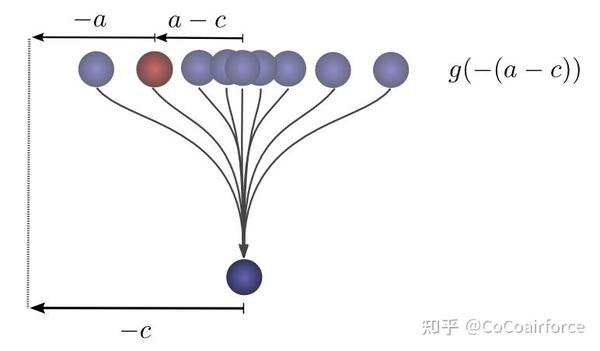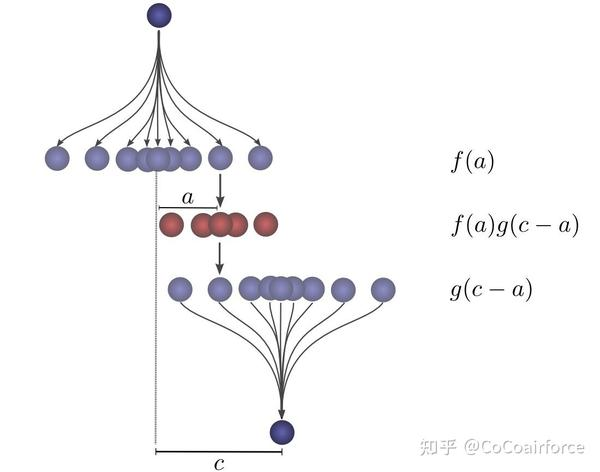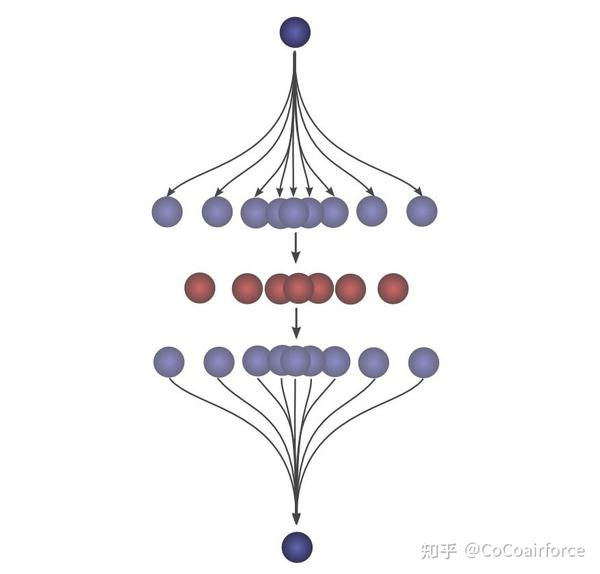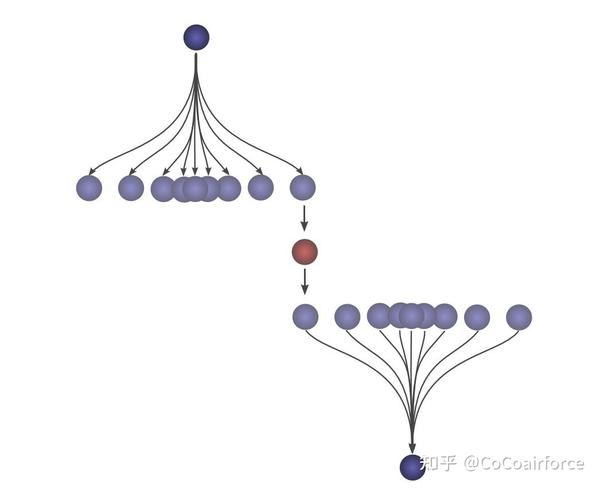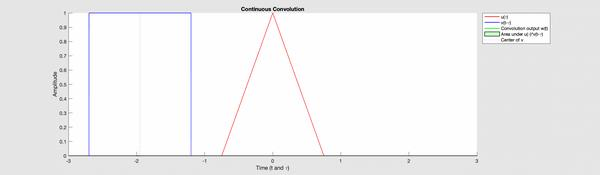## 高维卷积--Higher Dimensional Convolutions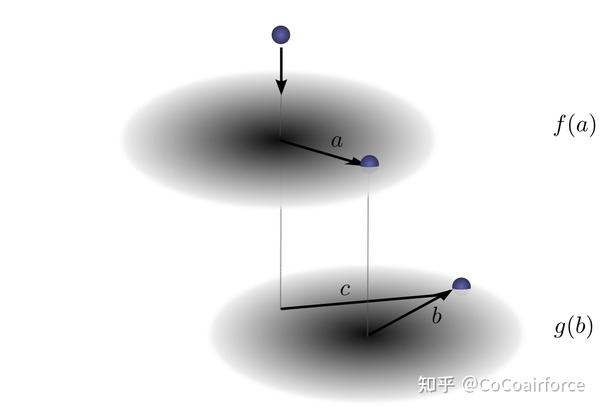$$(f * g)(c)=\sum_{a+b=c} f(a) \cdot g(b)$$

$$(f * g)\left(c{1}, c{2}\right)=\sum{a{1}+b{1}=c{1} \atop a{2}+b{2}=c{2}} f\left(a{1}, a{2}\right) \cdot g\left(b{1}, b_{2}\right)$$

$$(f * g)\left(c{1}, c{2}\right)=\sum{a{1}, a{2}} f\left(a{1}, a{2}\right) \cdot g\left(c{1}-a{1}, c{2}-a_{2}\right)$$

## 卷积神经网络--Convolutional Neural Networks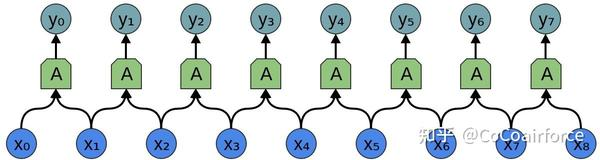• $y=A \cdot x$

$x$ 是输入信号，$y$是输出信号，$A$ 是系统，这个系统由 $n$ 个神经元组成，可以用输入来描述输出。

• $y = \left(W x +b\right)$
• 其中 $x =\left{x{1},x{2},\cdots ,x{n}\right}$是输入,$W =\left{w{1},w{2},\cdots ,w{n}\right}$ 是权重。权重描述了神经元与输入的连接方式。
• 负的权重意味着输入会抑制神经元发射，而正的权重则鼓励它发射。
• 权重是神经元的心脏，控制着它的行为.如果说2个神经元是相同的，即它们的权重是相同的。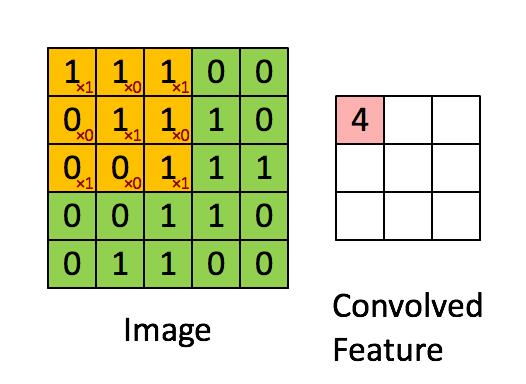Kernel 以一定的步伐向右移动，直到它解析出整行的宽度。接着，它以相同的步伐值跳到图像的开头（左边），并重复这个过程，直到遍历整个图像。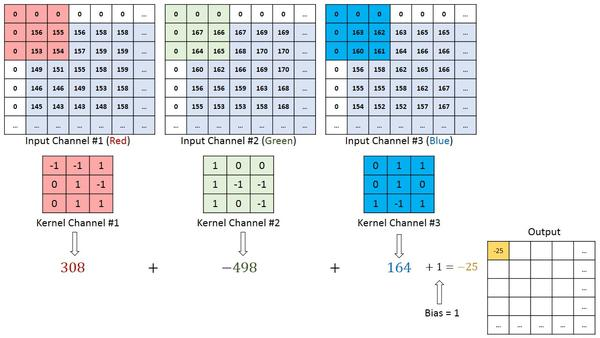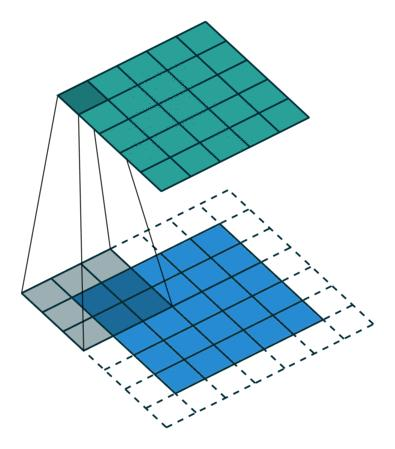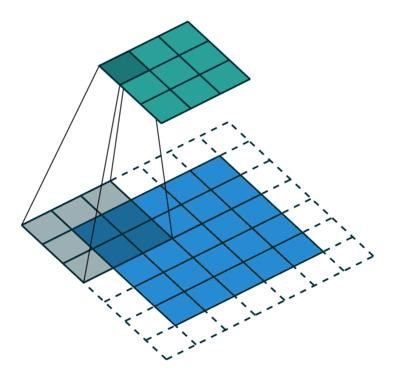## 池化层 -- Pooling Layer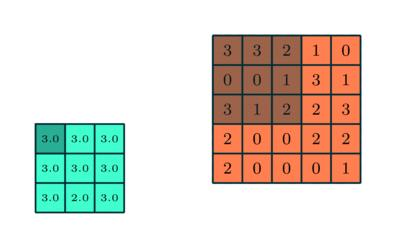Max Pooling也是一种噪声抑制器。它完全丢弃了嘈杂的激活，并在降低维度的同时进行去噪。另一方面，Average Pooling只是作为噪声抑制机制进行维度降低。因此，我们可以说Max Pooling的性能比Average Pooling好很多。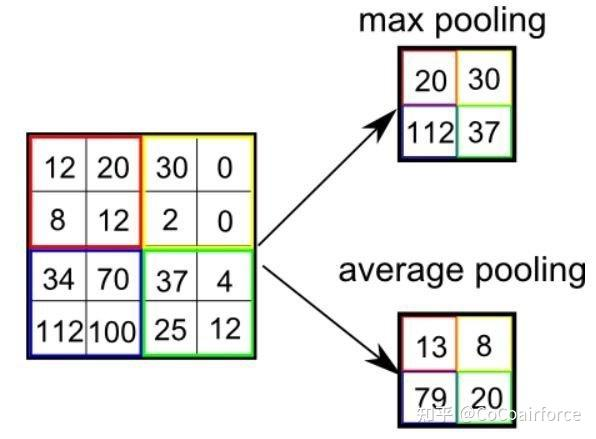## 全连接层(FC层) —- Fully Connected Layer (FC Layer)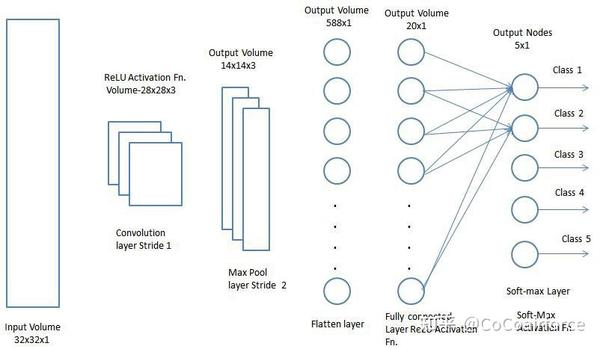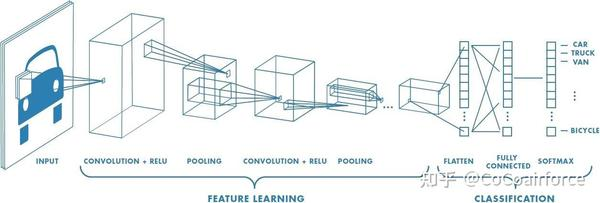## 总结 -- Conclusion

• 第一个好处是，我们有了一些非常强大的语言来描述神经网络的层。卷积大大简化了繁琐的计算工作。
• 其次，卷积非常容易实现。现存的许多库都提供了高效的卷积方法。
• 此外，卷积看起来是一个$O\left(n^{2}\right)$ 操作，但使用一些相当深刻的数学见解，可以创建一个 $O(n \log (n))$ 的实现。

Tips:

$$\sum{a+b=c} f(a) \cdot g(b)=\sum{b+a=c} g(b) \cdot f(a)$$

$$\sum{(a+b)+c=d}(f(a) \cdot g(b)) \cdot h(c)=\sum{a+(b+c)=d} f(a) \cdot(g(b) \cdot h(c))$$